RS Aggarwal Class 8 Solutions Chapter 3 - Squares And Square Roots

RS Aggarwal Class 8 Chapter 3 - Squares And Square Roots Solutions Free PDF

This chapter is designed to teach students about the square and square roots. After solving the question from this chapter the students will know to find the square and square roots of a given number. To practice more questions based on square and square roots, students are advised to solve the RS Aggarwal for class 8. For better preparation and practice students can also refer to RS Aggarwal class 8 solutions chapter 3 square and square roots.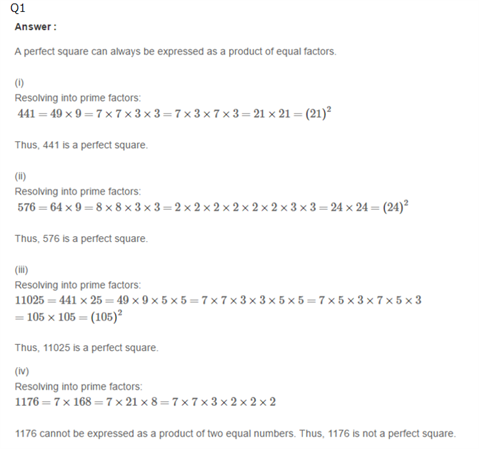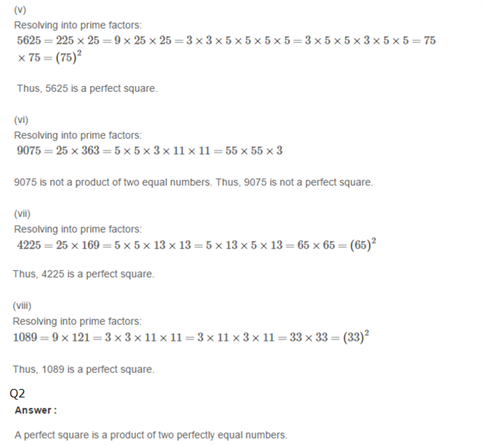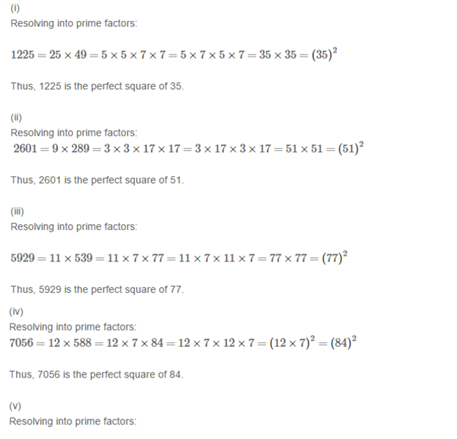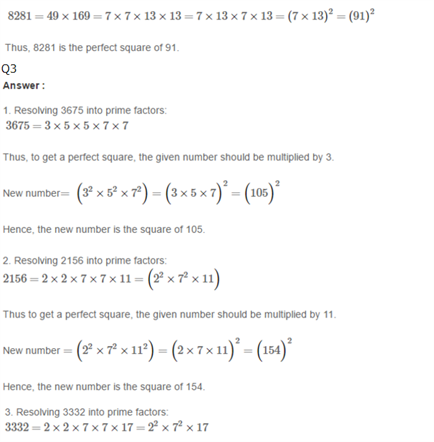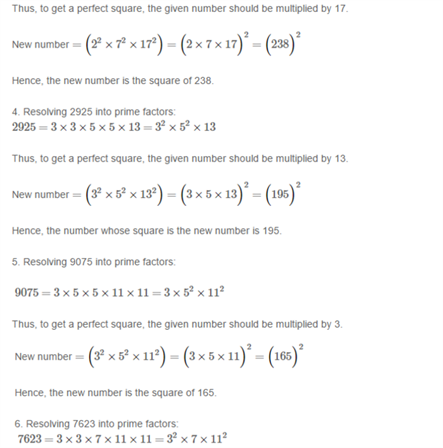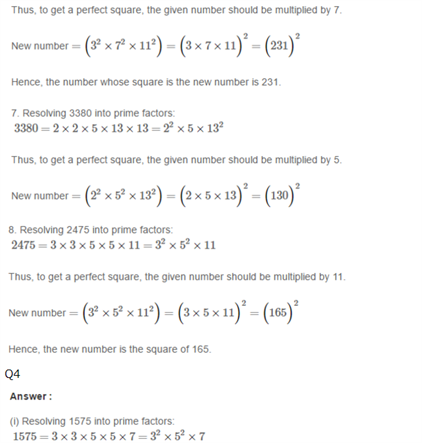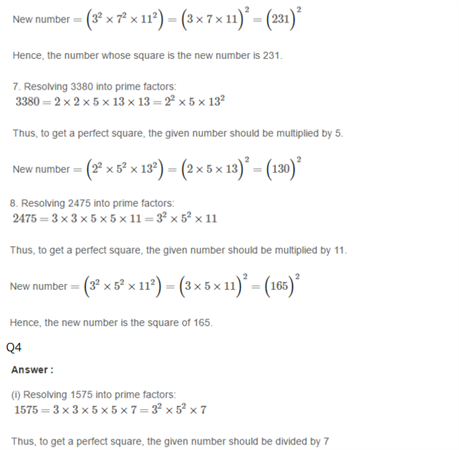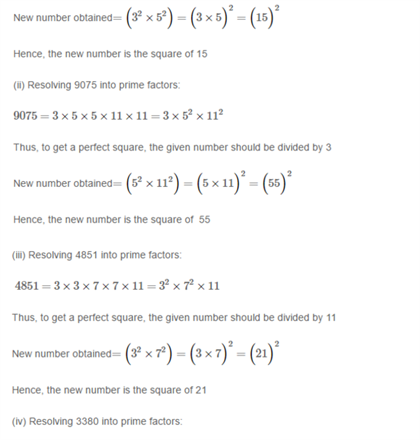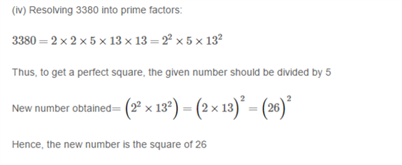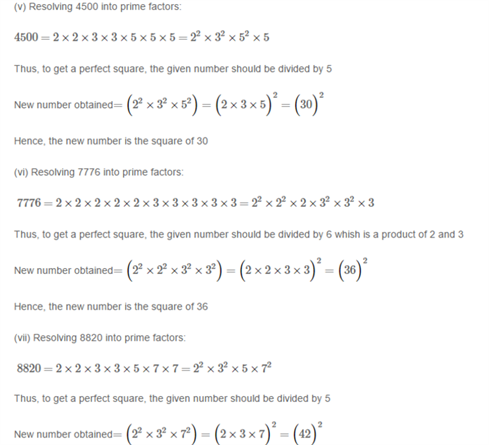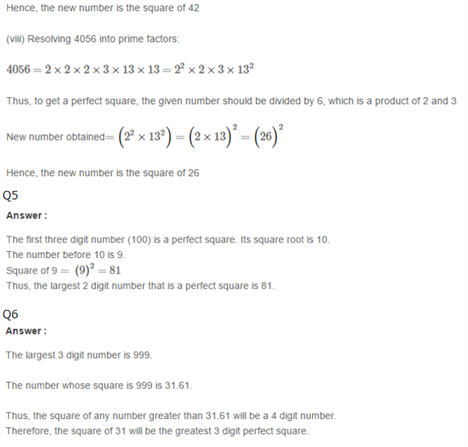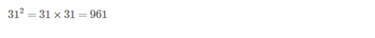Practise This Question

Can we precisely predict the occurrence of an earthquake using the latest technology?